# Coulomb's law

Coulomb's law or Coulomb's inverse-square law, is a law of physics that describes force interacting between static electrically charged particles. In its scalar form the law is:,

where ke is Coulomb's constant (ke = 8.99×109 N m2 C−2), q1 and q2 are the signed magnitudes of the charges, and the scalar r is the distance between the charges. The force of interaction between the charges is attractive if the charges have opposite signs (i.e. F is negative) and repulsive if like-signed (i.e. F is positive).

The law was first published in 1784 by French physicist Charles Augustin de Coulomb and was essential to the development of the theory of electromagnetism. It is analogous to Isaac Newton's inverse-square law of universal gravitation. Coulomb's law can be used to derive Gauss's law, and vice versa. The law has been tested extensively, and all observations have upheld the law's principle.

## History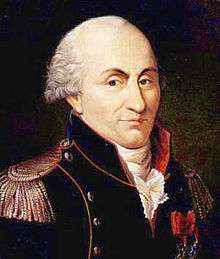Charles-Augustin de Coulomb

Ancient cultures around the Mediterranean knew that certain objects, such as rods of amber, could be rubbed with cat's fur to attract light objects like feathers. Thales of Miletus made a series of observations on static electricity around 600 BC, from which he believed that friction rendered amber magnetic, in contrast to minerals such as magnetite, which needed no rubbing. Thales was incorrect in believing the attraction was due to a magnetic effect, but later science would prove a link between magnetism and electricity. Electricity would remain little more than an intellectual curiosity for millennia until 1600, when the English scientist William Gilbert made a careful study of electricity and magnetism, distinguishing the lodestone effect from static electricity produced by rubbing amber. He coined the New Latin word electricus ("of amber" or "like amber", from ἤλεκτρον [elektron], the Greek word for "amber") to refer to the property of attracting small objects after being rubbed. This association gave rise to the English words "electric" and "electricity", which made their first appearance in print in Thomas Browne's Pseudodoxia Epidemica of 1646.

Early investigators of the 18th century who suspected that the electrical force diminished with distance as the force of gravity did (i.e., as the inverse square of the distance) included Daniel Bernoulli and Alessandro Volta, both of whom measured the force between plates of a capacitor, and Franz Aepinus who supposed the inverse-square law in 1758.

Based on experiments with electrically charged spheres, Joseph Priestley of England was among the first to propose that electrical force followed an inverse-square law, similar to Newton's law of universal gravitation. However, he did not generalize or elaborate on this. In 1767, he conjectured that the force between charges varied as the inverse square of the distance.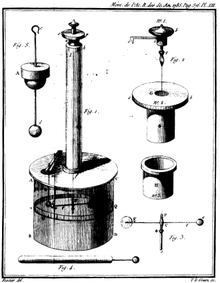Coulomb’s torsion balance

In 1769, Scottish physicist John Robison announced that, according to his measurements, the force of repulsion between two spheres with charges of the same sign varied as x−2.06.

In the early 1770s, the dependence of the force between charged bodies upon both distance and charge had already been discovered, but not published, by Henry Cavendish of England.

Finally, in 1785, the French physicist Charles-Augustin de Coulomb published his first three reports of electricity and magnetism where he stated his law. This publication was essential to the development of the theory of electromagnetism. He used a torsion balance to study the repulsion and attraction forces of charged particles, and determined that the magnitude of the electric force between two point charges is directly proportional to the product of the charges and inversely proportional to the square of the distance between them.

The torsion balance consists of a bar suspended from its middle by a thin fiber. The fiber acts as a very weak torsion spring. In Coulomb's experiment, the torsion balance was an insulating rod with a metal-coated ball attached to one end, suspended by a silk thread. The ball was charged with a known charge of static electricity, and a second charged ball of the same polarity was brought near it. The two charged balls repelled one another, twisting the fiber through a certain angle, which could be read from a scale on the instrument. By knowing how much force it took to twist the fiber through a given angle, Coulomb was able to calculate the force between the balls and derive his inverse-square proportionality law.

## The law

Coulomb's law states that:

The magnitude of the electrostatic force of interaction between two point charges is directly proportional to the scalar multiplication of the magnitudes of charges and inversely proportional to the square of the distance between them.

The force is along the straight line joining them. If the two charges have the same sign, the electrostatic force between them is repulsive; if they have different signs, the force between them is attractive.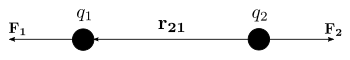Coulomb's law can also be stated as a simple mathematical expression. The scalar and vector forms of the mathematical equation areandrespectively,

where ke is Coulomb's constant (ke = 8.9875517873681764×109 N m2 C−2), q1 and q2 are the signed magnitudes of the charges, the scalar r is the distance between the charges, the vector r21 = r1r2 is the vectorial distance between the charges, and 21 = r21/|r21| (a unit vector pointing from q2 to q1). The vector form of the equation calculates the force F1 applied on q1 by q2. If r12 is used instead, then the effect on q2 can be found. It can be also calculated using Newton's third law: F2 = −F1.

### Units

When the electromagnetic theory is expressed using the standard SI units, force is measured in newtons, charge in coulombs, and distance in metres. Coulomb's constant is given by ke = 1ε0. The constant ε0 is the permittivity of free space in C2 m−2 N−1. And ε is the relative permittivity of the material in which the charges are immersed, and is dimensionless.

The SI derived units for the electric field are volts per meter, newtons per coulomb, or tesla meters per second.

Coulomb's law and Coulomb's constant can also be interpreted in various terms:

Cgs units are often preferred in the treatment of electromagnetism, as they greatly simplify formulas.

### Electric field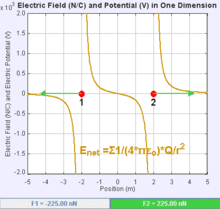If two charges have the same sign, the electrostatic force between them is repulsive; if they have different sign, the force between them is attractive.

An electric field is a vector field that associates to each point in space the Coulomb force experienced by a test charge. In the simplest case, the field is considered to be generated solely by a single source point charge. The strength and direction of the Coulomb force F on a test charge qt depends on the electric field E that it finds itself in, such that F = qtE. If the field is generated by a positive source point charge q, the direction of the electric field points along lines directed radially outwards from it, i.e. in the direction that a positive point test charge qt would move if placed in the field. For a negative point source charge, the direction is radially inwards.

The magnitude of the electric field E can be derived from Coulomb's law. By choosing one of the point charges to be the source, and the other to be the test charge, it follows from Coulomb's law that the magnitude of the electric field E created by a single source point charge q at a certain distance from it r in vacuum is given by:### Coulomb's constant

Main article: Coulomb's constant

Coulomb's constant is a proportionality factor that appears in Coulomb's law as well as in other electric-related formulas. Denoted ke, it is also called the electric force constant or electrostatic constant, hence the subscript e.

The exact value of Coulomb's constant is:### Conditions for validity

There are three conditions to be fulfilled for the validity of Coulomb’s law:

1. The charges must have a spherically symmetric distribution (e.g. be point charges, or a charged metal sphere.
2. The charges must not overlap (e.g. be distinct point charges)
3. The charges must be stationary with respect to each other.

## Scalar form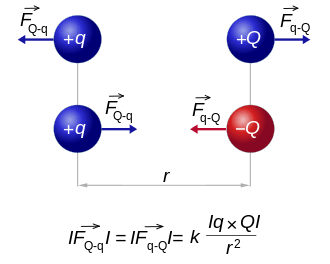The absolute value of the force F between two point charges q and Q relates to the distance between the point charges and to the simple product of their charges. The diagram shows that like charges repel each other, and opposite charges attract each other.

When it is only of interest to know the magnitude of the electrostatic force (and not its direction), it may be easiest to consider a scalar version of the law. The scalar form of Coulomb's Law relates the magnitude and sign of the electrostatic force F acting simultaneously on two point charges q1 and q2 as follows:where r is the separation distance and ke is Coulomb's constant. If the product q1q2 is positive, the force between the two charges is repulsive; if the product is negative, the force between them is attractive.

## Vector formIn the image, the vector F1 is the force experienced by q1, and the vector F2 is the force experienced by q2. When q1q2 > 0 the forces are repulsive (as in the image) and when q1q2 < 0 the forces are attractive (opposite to the image). The magnitude of the forces will always be equal.

Coulomb's law states that the electrostatic force F1 experienced by a charge, q1 at position r1, in the vicinity of another charge, q2 at position r2, in a vacuum is equal to:where r21 = r1r2, the unit vector 21 = r21/|r21|, and ε0 is the electric constant.

The vector form of Coulomb's law is simply the scalar definition of the law with the direction given by the unit vector, 21, parallel with the line from charge q2 to charge q1. If both charges have the same sign (like charges) then the product q1q2 is positive and the direction of the force on q1 is given by 21; the charges repel each other. If the charges have opposite signs then the product q1q2 is negative and the direction of the force on q1 is given by 21 = 12; the charges attract each other.

The electrostatic force F2 experienced by q2, according to Newton's third law, is F2 = −F1.

### System of discrete charges

The law of superposition allows Coulomb's law to be extended to include any number of point charges. The force acting on a point charge due to a system of point charges is simply the vector addition of the individual forces acting alone on that point charge due to each one of the charges. The resulting force vector is parallel to the electric field vector at that point, with that point charge removed.

The force F on a small charge q at position r, due to a system of N discrete charges in vacuum is:where qi and ri are the magnitude and position respectively of the ith charge, i is a unit vector in the direction of Ri = rri (a vector pointing from charges qi to q).

### Continuous charge distribution

In this case, the principle of linear superposition is also used. For a continuous charge distribution, an integral over the region containing the charge is equivalent to an infinite summation, treating each infinitesimal element of space as a point charge dq. The distribution of charge is usually linear, surface or volumetric.

For a linear charge distribution (a good approximation for charge in a wire) where λ(r′) gives the charge per unit length at position r′, and dl′ is an infinitesimal element of length,.

For a surface charge distribution (a good approximation for charge on a plate in a parallel plate capacitor) where σ(r′) gives the charge per unit area at position r′, and dA′ is an infinitesimal element of area,For a volume charge distribution (such as charge within a bulk metal) where ρ(r′) gives the charge per unit volume at position r′, and dV′ is an infinitesimal element of volume,The force on a small test charge q′ at position r in vacuum is given by the integral over the distribution of charge:## Simple experiment to verify Coulomb's law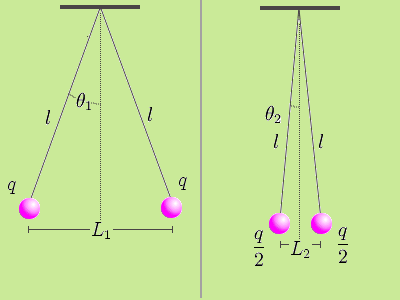It is possible to verify Coulomb's law with a simple experiment. Consider two small spheres of mass m and same-sign charge q, hanging from two ropes of negligible mass of length l. The forces acting on each sphere are three: the weight mg, the rope tension T and the electric force F.

In the equilibrium state:(1)

and:(2)

Dividing (1) by (2):(3)

Let L1 be the distance between the charged spheres; the repulsion force between them F1, assuming Coulomb's law is correct, is equal to(Coulomb's law)

so:(4)

If we now discharge one of the spheres, and we put it in contact with the charged sphere, each one of them acquires a charge q/2. In the equilibrium state, the distance between the charges will be L2 < L1 and the repulsion force between them will be:(5)

We know that F2 = mg tan θ2. And:Dividing (4) by (5), we get:(6)

Measuring the angles θ1 and θ2 and the distance between the charges L1 and L2 is sufficient to verify that the equality is true taking into account the experimental error. In practice, angles can be difficult to measure, so if the length of the ropes is sufficiently great, the angles will be small enough to make the following approximation:(7)

Using this approximation, the relationship (6) becomes the much simpler expression:(8)

In this way, the verification is limited to measuring the distance between the charges and check that the division approximates the theoretical value.

## Electrostatic approximation

In either formulation, Coulomb’s law is fully accurate only when the objects are stationary, and remains approximately correct only for slow movement. These conditions are collectively known as the electrostatic approximation. When movement takes place, magnetic fields that alter the force on the two objects are produced. The magnetic interaction between moving charges may be thought of as a manifestation of the force from the electrostatic field but with Einstein’s theory of relativity taken into consideration.

### Atomic forces

Coulomb's law holds even within atoms, correctly describing the force between the positively charged atomic nucleus and each of the negatively charged electrons. This simple law also correctly accounts for the forces that bind atoms together to form molecules and for the forces that bind atoms and molecules together to form solids and liquids. Generally, as the distance between ions increases, the energy of attraction approaches zero and ionic bonding is less favorable. As the magnitude of opposing charges increases, energy increases and ionic bonding is more favorable.

## Notes

1. Stewart, Joseph (2001). Intermediate Electromagnetic Theory. World Scientific. p. 50. ISBN 981-02-4471-1
2. Simpson, Brian (2003). Electrical Stimulation and the Relief of Pain. Elsevier Health Sciences. pp. 6–7. ISBN 0-444-51258-6
3. Baigrie, Brian (2006). Electricity and Magnetism: A Historical Perspective. Greenwood Press. pp. 7–8. ISBN 0-313-33358-0
4. Chalmers, Gordon (1937). "The Lodestone and the Understanding of Matter in Seventeenth Century England". Philosophy of Science. 4 (1): 75–95. doi:10.1086/286445
5. Socin, Abel (1760). Acta Helvetica Physico-Mathematico-Anatomico-Botanico-Medica (in Latin). 4. Basileae. pp. 224, 225.
6. Heilbron, J.L. (1979). Electricity in the 17th and 18th Centuries: A Study of Early Modern Physics. Los Angeles, California: University of California Press. pp. 460–462 and 464 (including footnote 44). ISBN 0486406881.
7. Schofield, Robert E. (1997). The Enlightenment of Joseph Priestley: A Study of his Life and Work from 1733 to 1773. University Park: Pennsylvania State University Press. pp. 144–56. ISBN 0-271-01662-0.
8. Priestley, Joseph (1767). The History and Present State of Electricity, with Original Experiments. London, England. p. 732.
May we not infer from this experiment, that the attraction of electricity is subject to the same laws with that of gravitation, and is therefore according to the squares of the distances; since it is easily demonstrated, that were the earth in the form of a shell, a body in the inside of it would not be attracted to one side more than another?
9. Elliott, Robert S. (1999). Electromagnetics: History, Theory, and Applications. ISBN 978-0-7803-5384-8.
10. Robison, John (1822). Murray, John, ed. A System of Mechanical Philosophy. 4. London, England.
On page 68, the author states that in 1769 he announced his findings regarding the force between spheres of like charge. On page 73, the author states the force between spheres of like charge varies as x−2.06:
The result of the whole was, that the mutual repulsion of two spheres, electrified positively or negatively, was very nearly in the inverse proportion of the squares of the distances of their centres, or rather in a proportion somewhat greater, approaching to x−2.06.
When making experiments with charged spheres of opposite charge the results were similar, as stated on page 73:
When the experiments were repeated with balls having opposite electricities, and which therefore attracted each other, the results were not altogether so regular and a few irregularities amounted to 16 of the whole; but these anomalies were as often on one side of the medium as on the other. This series of experiments gave a result which deviated as little as the former (or rather less) from the inverse duplicate ratio of the distances; but the deviation was in defect as the other was in excess.
Nonetheless, on page 74 the author infers that the actual action is related exactly to the inverse duplicate of the distance:
We therefore think that it may be concluded, that the action between two spheres is exactly in the inverse duplicate ratio of the distance of their centres, and that this difference between the observed attractions and repulsions is owing to some unperceived cause in the form of the experiment.
On page 75, the authour compares the electric and gravitational forces:
Therefore we may conclude, that the law of electric attraction and repulsion is similar to that of gravitation, and that each of those forces diminishes in the same proportion that the square of the distance between the particles increases.
11. Maxwell, James Clerk, ed. (1967) . "Experiments on Electricity: Experimental determination of the law of electric force.". The Electrical Researches of the Honourable Henry Cavendish... (1st ed.). Cambridge, England: Cambridge University Press. pp. 104–113.
On pages 111 and 112 the author states:
We may therefore conclude that the electric attraction and repulsion must be inversely as some power of the distance between that of the 2 + 150 th and that of the 2 − 150 th, and there is no reason to think that it differs at all from the inverse duplicate ratio.
12. Coulomb (1785a) "Premier mémoire sur l’électricité et le magnétisme," Histoire de l’Académie Royale des Sciences, pages 569-577 — Coulomb studied the repulsive force between bodies having electrical charges of the same sign:
Il résulte donc de ces trois essais, que l'action répulsive que les deux balles électrifées de la même nature d'électricité exercent l'une sur l'autre, suit la raison inverse du carré des distances. Translation: It follows therefore from these three tests, that the repulsive force that the two balls — [that were] electrified with the same kind of electricity — exert on each other, follows the inverse proportion of the square of the distance.
Coulomb also showed that oppositely charged bodies obey an inverse-square law of attraction.
13. Jackson, J. D. (1998) . Classical Electrodynamics (3rd ed.). New York: John Wiley & Sons. ISBN 978-0-471-30932-1. OCLC 535998.
14. Coulomb's law, Hyperphysics
15. Coulomb's law, University of Texas
16. Charged rods, PhysicsLab.org

ke = H/m is not correct it must be F/m

## References

• Coulomb, Charles Augustin (1788) . "Premier mémoire sur l'électricité et le magnétisme". Histoire de l’Académie Royale des Sciences. Imprimerie Royale. pp. 569–577.
• Coulomb, Charles Augustin (1788) . "Second mémoire sur l'électricité et le magnétisme". Histoire de l’Académie Royale des Sciences. Imprimerie Royale. pp. 578–611.
• Griffiths, David J. (1998). Introduction to Electrodynamics (3rd ed.). Prentice Hall. ISBN 0-13-805326-X.
• Tipler, Paul A.; Mosca, Gene (2008). Physics for Scientists and Engineers (6th ed.). New York: W. H. Freeman and Company. ISBN 0-7167-8964-7. LCCN 2007010418.
• Young, Hugh D.; Freedman, Roger A. (2010). Sears and Zemansky's University Physics : With Modern Physics (13th ed.). Addison-Wesley (Pearson). ISBN 978-0-321-69686-1.